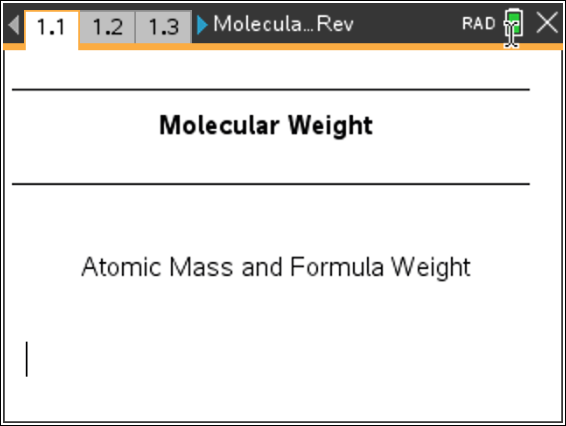# Activities

•##### Download

•• ##### Subject Area

• Science: Chemistry: Atomic Structure and Periodic Table

• ##### Author9-12

45 Minutes

• ##### Device
• TI-Nspire™ Navigator™
• TI-Nspire™
• TI-Nspire™ CAS
•TI-Nspire™ CX/CX II
• ##### Software

TI-Nspire™ CX
TI-Nspire™ CX CAS

4.5

## Molecular Weight#### Activity Overview

Students will find the atomic mass on the periodic table and use this to determine the mass of one atom, one mole of atoms. They will also determine formula masses.

#### Objectives

• Use the periodic table to determine atomic mass.
• Calculate the formula weight for molecules.

#### About the Lesson

The following questions will guide student exploration during this activity:

• What is a mole?
• Which units are used for the mass of atoms, and which units are used for the mass of moles of atoms?
• How do you determine the number of atoms of each element in a molecule?

• The goal of this activity is for students to explore the concepts of atomic mass and formula weight. Students will use the periodic table to determine the atomic mass of elements and then use that information to calculate the molecular weight for various molecules.